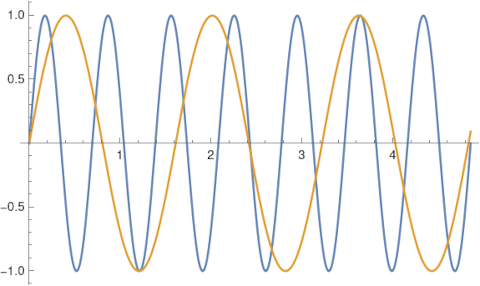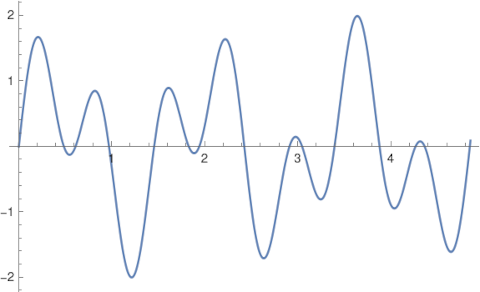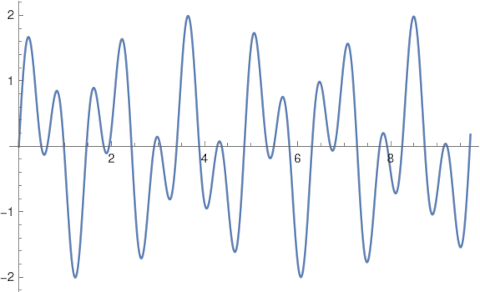# 2s, 5s, and decibels

Last night I ran across a delightful Twitter thread by Nathan Mishra-Linger that starts out by saying

It’s nice that 2^7 ≈ 5^3, because that lets you approximate any integer valued decibel quantity as a product of powers of 2 and 5.

The observation that

27 ≈ 53,

is more familiar if you multiply both sides by 23. That is,

210 ≈ 103

This is the basis of using “kilo” to mean both 1000 and 1024 in different contexts. More on that here.

## Decibel approximations

Nathan points out that this happy coincidence means that small integer decibels can be written as small powers of 2 and 5. Here’s a table based on one of the tweets in Nathan’s thread.

0 dB = 100.0 = 2050
1 dB = 100.1 ≈ 2-251
2 dB = 100.2 ≈ 235-1
3 dB = 100.3 ≈ 2150
4 dB = 100.4 ≈ 2-151
5 dB = 100.5 ≈ 2-352
6 dB = 100.6 ≈ 2250
7 dB = 100.7 ≈ 2051
8 dB = 100.8 ≈ 255-1
9 dB = 100.9 ≈ 2350

You can show that the maximum relative error above is 1.4% with the following Python code.

```    approx = [
2** 0 * 5** 0,
2**-2 * 5** 1,
2** 3 * 5**-1,
2** 1 * 5** 0,
2**-1 * 5** 1,
2**-3 * 5** 2,
2** 2 * 5** 0,
2** 0 * 5** 1,
2** 5 * 5**-1,
2** 3 * 5** 0,
]

err = [abs(1 - approx[n]/10**(n/10)) for n in range(10)]
print(max(err))
```

## Almost in step

The last tweet in Nathan’s thread is a diagram showing how powers of 2 and 5 sort of cycle. Here’s another to look at that.

Taking logs of both sides of

27 ≈ 53,

shows that

7 log 2 ≈ 3 log 5.

Suppose two people start out walking together, one taking lots of small steps and the other taking fewer larger steps. Specifically, one takes 7 steps of size log 2 in the time that it takes the other to make 3 step of size log 5. Then periodically they’ll almost be in step.

This reminded me of my recent thread about how Earth and Mars line up approximately every 17 years.

Suppose you have two planets orbiting a star. One takes time log 2 to orbit, and another one, further from the star, takes time log 5 to orbit. Then after the inner planet takes 7 orbits and the outer planet takes 3, the two planets will be approximately in a straight line with their star.

## Plots

One last observation. Above I said “periodically they’ll almost be in step.” There’s a rigorous way to use the words “almost” and “periodic.” The sum of a sine wave of period log 2 and a sine wave of period log 5 is an “almost periodic” function. See my next post for the rigorous definition of an almost periodic function, but for now I’ll just include a few plots that the function described in this paragraph is “almost periodic” in the colloquial sense.

Here’s a plot of two sine waves, one with 7 periods of length log 2 and the other with 3 periods of length 5.

`    Plot[{Sin[2 Pi t/Log], Sin[2 Pi t/Log]}, {t, 0, 7 Log }]`Here’s their sum.

`    Plot[Sin[2 Pi t/Log] + Sin[2 Pi t/Log], {t, 0, 7 Log }]`And here’s two approximate periods of the sum.

`    Plot[Sin[2 Pi t/Log] + Sin[2 Pi t/Log], {t, 0, 14 Log }]`## 3 thoughts on “2s, 5s, and decibels”

1. Jonathan

I feel like the fact that 3 and 7 are relatively prime should also be included in the observation.

2. The proximity of 2^^7 and 5^^3 is also the basis of many of the perennial disputes over musical temperament. See my paper Tertium Quid.

3. Juan José Alba González

It’s odd that for 8 Db you choose 2^5 * 5^-1 instead of the better and simpler approximation 2^-2 5^2. That reduces the maximum relative error from 1.4% to 1.1%